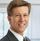## Distribution function of a linear combination of chi-squares

Started ‎12-15-2014 by
Modified ‎04-04-2022 by
Views 1,877

An IML module is provided for calculating the distribution function and the density of a positive linear combination of independent central chi-square variates. The cumulative distribution function (CDF) is obtained by inverting the moment generating function as described in the paper by Moschopoulos and Canada: "The distribution function of a linear combination of chi-squares" (Comp. and Maths. with Appls., Vol.10, Nº 4/5, pp 383-386, 1984) . The distribution function is expressed as an infinite series of gamma distribution functions. Similarly, the density (PDF) can be expressed as an infinite series of gamma densities. The number of terms of the series used in calculating the CDF and the PDF should be specified by the user. An upper limit of the truncation error is provided for both, PDF and CDF. Optionally, the CDF may be estimated by simulation.Nice work.  Notice that you can also get a finite-difference approximation to the PDF. Here is a visualization of the first example in the program:

delta = 0.01;

quant = do(0,3,delta);

run sum_chisq(prob,coef,df,quant);

title2 "CDF";

call series(quant, prob);

title2 "PDF";

pdf = dif(prob`)/delta;

call series(quant, pdf);Thanks Rick,

I like the graphical presentations and the simple series subroutine. The PDF can also be calculated by replacing the CDF of the gamma distribution in the module by the corresponding PDF. The numerical differentiation that you supplied was a valuable check.

Regards

Version history
Last update:
‎04-04-2022 03:12 PM
Updated by:Contributors
Article Labels
Article Tags